Courses

# Calculus (Basic Level) - 1

## 10 Questions MCQ Test Mock Test Series for Electrical Engineering (EE) GATE 2020 | Calculus (Basic Level) - 1

Description
This mock test of Calculus (Basic Level) - 1 for GATE helps you for every GATE entrance exam. This contains 10 Multiple Choice Questions for GATE Calculus (Basic Level) - 1 (mcq) to study with solutions a complete question bank. The solved questions answers in this Calculus (Basic Level) - 1 quiz give you a good mix of easy questions and tough questions. GATE students definitely take this Calculus (Basic Level) - 1 exercise for a better result in the exam. You can find other Calculus (Basic Level) - 1 extra questions, long questions & short questions for GATE on EduRev as well by searching above.
QUESTION: 1

### Consider the function y = | x | in the interval [-1, 1], In this interval, the function is

Solution:

The function y = | x | in the interval [-1, 1 ] is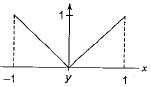| x | is continuous and differentiable every where except at x = 0, where it is continuous but not differentiable.
Since [-1, 1] contains 0, in this interval it is continuous but not differentiable.

QUESTION: 2

###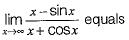Solution: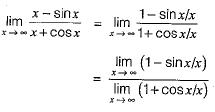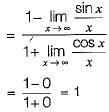QUESTION: 3

### What is the value of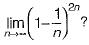Solution: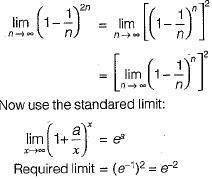QUESTION: 4

Which one of the following functions is continuous at x = 3?

Solution: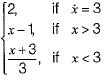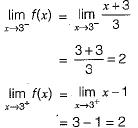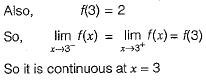QUESTION: 5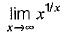is

Solution: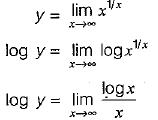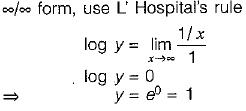QUESTION: 6

Evaluate the limit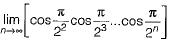Solution: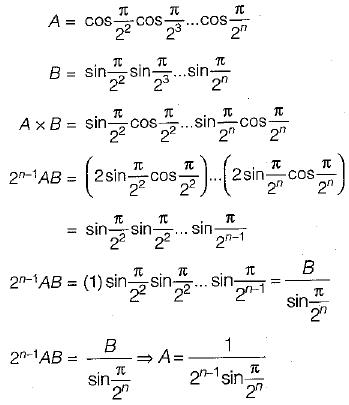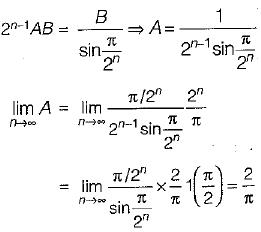QUESTION: 7

The maximum value of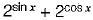Solution: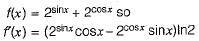to find the maximum it is enough to consider for [ ]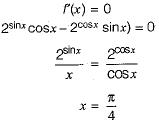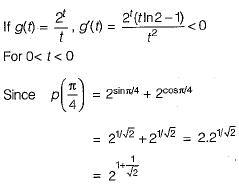QUESTION: 8

A polynomial p(x) is such that p(0) = 5, p(1) = 4, p(2) = 9 and p(3) = 20. The minimum degree it can have is

Solution:

Let, P(x) is of degree 1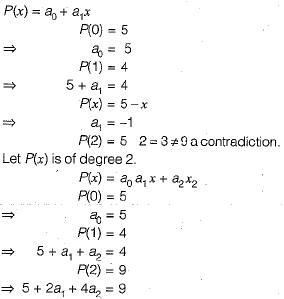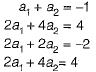SubtractQUESTION: 9

The formula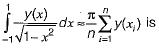Solution:

This is Gauss-Chebyshev formula.

QUESTION: 10

Which of the methods is used for finding solutions of differential equations?

Solution:

Euler method is used for solution of differential equation.